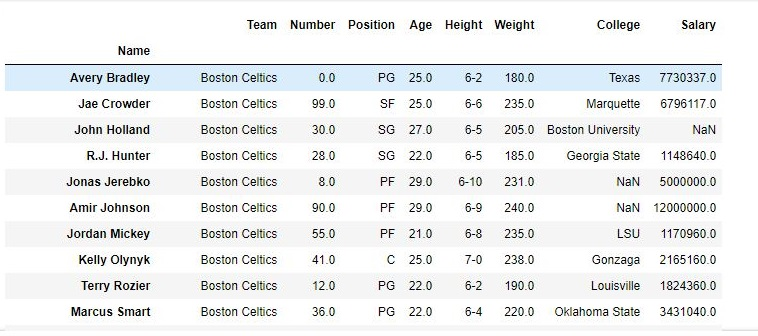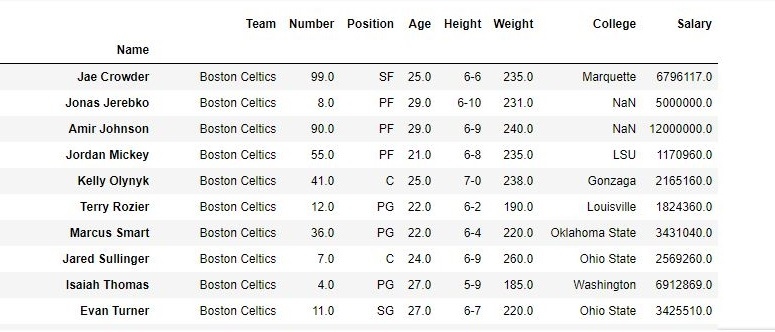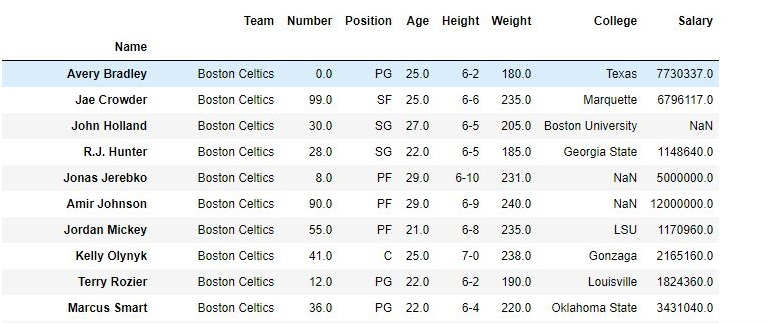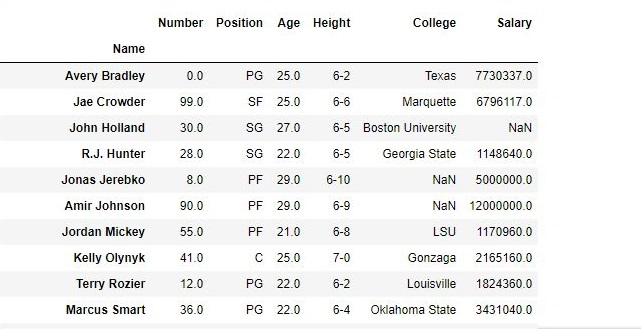# Python – Delete rows/columns from DataFrame using Pandas.drop()

## Python | Delete rows/columns from DataFrame using Pandas.drop()

Python is a great language for doing data analysis, primarily because of the fantastic ecosystem of data-centric Python packages. Pandas is one of those packages and makes importing and analyzing data much easier.

Pandas provide data analysts a way to delete and filter data frame using `.drop()` method. Rows or columns can be removed using index label or column name using this method.

Syntax:
DataFrame.drop(labels=None, axis=0, index=None, columns=None, level=None, inplace=False, errors=’raise’)

Parameters:

labels: String or list of strings referring row or column name.
axis: int or string value, 0 ‘index’ for Rows and 1 ‘columns’ for Columns.
index or columns: Single label or list. index or columns are an alternative to axis and cannot be used together.
level: Used to specify level in case data frame is having multiple level index.
inplace: Makes changes in original Data Frame if True.
errors: Ignores error if any value from the list doesn’t exists and drops rest of the values when errors = ‘ignore’

Return type: Dataframe with dropped values

Example #1: Dropping Rows by index label
In his code, A list of index labels is passed and the rows corresponding to those labels are dropped using .drop() method.

 `# importing pandas module` `import` `pandas as pd` ` ` `# making data frame from csv file` `data ``=` `pd.read_csv(``"nba.csv"``, index_col ``=``"Name"` `)` ` ` `# dropping passed values` `data.drop([``"Avery Bradley"``, ``"John Holland"``, ``"R.J. Hunter"``,` `                            ``"R.J. Hunter"``], inplace ``=` `True``)` ` ` `# display` `data`

Output:
As shown in the output images, the new output doesn’t have the passed values. Those values were dropped and the changes were made in the original data frame since inplace was True.

Data Frame before Dropping values-Data Frame after Dropping values-Example #2 : Dropping columns with column name

In his code, Passed columns are dropped using column names. `axis `parameter is kept 1 since 1 refers to columns.

 `# importing pandas module` `import` `pandas as pd` ` ` `# making data frame from csv file` `data ``=` `pd.read_csv(``"nba.csv"``, index_col ``=``"Name"` `)` ` ` `# dropping passed columns` `data.drop([``"Team"``, ``"Weight"``], axis ``=` `1``, inplace ``=` `True``)` ` ` `# display` `data`

Output:
As shown in the output images, the new output doesn’t have the passed columns. Those values were dropped since axis was set equal to 1 and the changes were made in the original data frame since inplace was True.

Data Frame before Dropping Columns-Data Frame after Dropping Columns-Last Updated on October 28, 2021 by admin

## Pandas Dataframe.duplicated()Pandas Dataframe.duplicated()

Pandas Dataframe.duplicated() Pandas is one of those packages and makes importing and analyzing data much

## String manipulations in Pandas DataFrameString manipulations in Pandas DataFrame

String manipulations in Pandas DataFrame String manipulation is the process of changing, parsing, splicing, pasting, or

Python | Pandas Series.str.pad() Python is a great language for doing data analysis, primarily because

## Python – Visualize missing values (NaN) values using Missingno LibraryPython – Visualize missing values (NaN) values using Missingno Library

Python | Visualize missing values (NaN) values using Missingno Library In the case of a

## Find location of an element in Pandas dataframe in PythonFind location of an element in Pandas dataframe in Python

Find location of an element in Pandas dataframe in Python In this article, we will

## Boolean Indexing in PandasBoolean Indexing in Pandas

In boolean indexing, we will select subsets of data based on the actual values of

## Merge two Pandas DataFrames based on closest DateTimeMerge two Pandas DataFrames based on closest DateTime

Merge two Pandas DataFrames based on closest DateTime In this article, we will discuss how